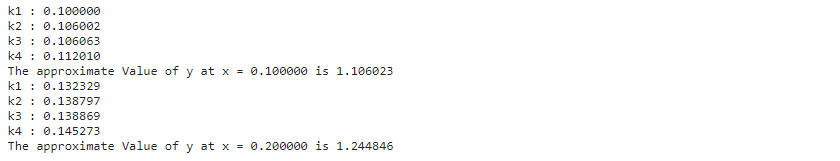In this section we will study about the Runge-Kutta Method. In the previous section, we learnt about Euler’s Method and came to a conclusion that the accuracy of the solution is compromised. Hence, in order to get better results of accuracy, we will learn about Runge-Kutta method.$\frac{\text{d}y}{\text{d}x}=f(x,y)$,                     and$y(x_{0})=y_{0}$$k_{1}=h$$f(x_{n},y_{n})$$k_{2}=h$$f(x_{n}+ \frac {h}{2},y_{n}+ \frac {h}{2} k_{1})$$k_{3}=h$$f(x_{n}+ \frac {h}{2},y_{n}+ \frac {h}{2} k_{2})$$k_{4}=h$$f(x_{n}+ h,y_{n}+ h$$k_{3})$

Where the increment based on the slope at the beginning of the interval is$k_{1}$, the increment based on the slope at the mid-point of the interval are$k_{2}$$k_{3}$ and the increment based on the slope at the end of the interval is$k_{4}$.

We define more parameters to obtain good accuracy. In Euler’s Method, the assumption of having same slop throughout in between two consecutive points led to the poor result of accuracy. In Runge-Kutta Method, we will have multiple points (defined as parameters) in between the two consecutive points which will ensure good accuracy and reduce the impact of constant slop between two consecutive points(as in Euler’s Method).

Finally, we calculate$y_{n+1}$ as follows:$y_{n+1}=y_{n}+ \frac {1}{6} (k_{1} + 2k_{2}+ 2k_{3}+ k_{4})$

We can say that Euler’s Method is the First Order Runge-Kutta Method Approach.

Example 1:  Apply Runge-Kutta Method of fourth order to find an approximate value of$'y'$ at$x=0.2$,     if$\frac{\text{d}y}{\text{d}x}=x+y^{2}$ where$y(0)=1$ in steps of$h=0.1$

SOLUTION:  Given,$f(x_{n},y_{n}) = x + y^{2}$$x_{0} = 0$$y_{0}=1$ and$h = 0.1$.

We know,$k_{1}=h$$f(x_{n},y_{n})$$k_{2}=h$$f(x_{n}+ \frac {h}{2},y_{n}+ \frac {h}{2} k_{1})$$k_{3}=h$$f(x_{n}+ \frac {h}{2},y_{n}+ \frac {h}{2} k_{2})$$k_{4}=h$$f(x_{n}+ h,y_{n}+ h$$k_{3})$$y_{n+1}=y_{n}+ \frac {1}{6} (k_{1} + 2k_{2}+ 2k_{3}+ k_{4})$

Using the above formula, we calculate the value of$k_{1}, k_{2}, k_{3}$ and$k_{4}$ for$y(0.1)$$k_{1}=h$$f(x_{0},y_{0}) = 0.1$$k_{2}=h$$f(x_{0}+ \frac {h}{2},y_{0}+ \frac {h}{2} k_{1}) = 0.106002$$k_{3}=h$$f(x_{0}+ \frac {h}{2},y_{0}+ \frac {h}{2} k_{2}) = 0.106063$$k_{4}=h$$f(x_{0}+ h,y_{0}+ h$$k_{3}) = 0.112010$

We get,$k_{1}= 0.1$$k_{2}=0.106002$$k_{3}=0.106063$ and$k_{4}=0.112010$$y_{1}=y_{0}+ \frac {1}{6} (k_{1} + 2k_{2}+ 2k_{3}+ k_{4})$ and$x_{1}= x_{0} + h$

Therefore, the value of$y_{1}=y(0.1)=1.106023$ where$x_{1} = 0.1$

Similarly, we will now repeat the procedure using the value of$y_{1}$ &$x_{1}$ and calculate the value of$k_{1}, k_{2}, k_{3}$ and$k_{4}$ for$y(0.2)$.

We get,$k_{1}= 0.132329$$k_{2}=0.138797$$k_{3}=0.138869$ and$k_{4}=0.145273$$y_{2}=y_{1}+ \frac {1}{6} (k_{1} + 2k_{2}+ 2k_{3}+ k_{4})$

Therefore, we get the approximate value as$y_{2}=y(0.2)=1.244846$

Code:

				
// Runge-Kutta Method
// Solving dy/dx = x + y^2

%use s2

// Defining function f(x,y) for this case
val f: BivariateRealFunction = object : AbstractBivariateRealFunction() {
override fun evaluate(x: Double, y: Double): Double {
return x + (y*y)
}
}

val x_at_0 = 0.0
val y_at_0 = 1.0
val h = 0.1

val k: BivariateRealFunction = object : AbstractBivariateRealFunction() {
override fun evaluate(h: Double, ft: Double): Double {
return h*ft
}
}

// y(n+1) = y(n) + (1/6)*(k1 + 2k2 + 2k3 + k4)
val Y_at_n_plus_1: BivariateRealFunction = object : AbstractBivariateRealFunction() {
override fun evaluate(y_at_n: Double, k_term: Double): Double {
return y_at_n + (1.0/6.0)*k_term
}
}

val h_term: BivariateRealFunction = object : AbstractBivariateRealFunction() {
override fun evaluate(h: Double, t: Double): Double {
return h*t
}
}
val xn: BivariateRealFunction = object : AbstractBivariateRealFunction() {
override fun evaluate(x_at_0: Double, h_term: Double): Double {
return x_at_0 + h_term
}
}

val steps = mutableListOf(0.0, 1.0)    //no. of steps = 2 as x=0.2 will be reached in 2 steps
var y_n = y_at_0
for (index in steps){
val h_n = h_term.evaluate(h,index)
val x_n = xn.evaluate(x_at_0,h_n)

// k1 = h*f(x_n,y_n)
val f_n_1 = f.evaluate(x_n,y_n)
val k1_n = k.evaluate(h,f_n_1)
println("k1 : %f".format(k1_n))

// k2 = h*f(x_n + h/2, y_n + (h/2)*k1)
var xi = x_n + (h/2)
var yi = y_n + (h/2)*k1_n
val f_n_2 = f.evaluate(xi,yi)
val k2_n = k.evaluate(h,f_n_2)
println("k2 : %f".format(k2_n))

// k3 = h*f(x_n + h/2, y_n + (h/2)*k2)
xi = x_n + (h/2)
yi = y_n + (h/2)*k2_n
val f_n_3 = f.evaluate(xi,yi)
val k3_n = k.evaluate(h,f_n_3)
println("k3 : %f".format(k3_n))

// k4 = h*f(x_n + h,y_n + h*k3)
xi = x_n + h
yi = y_n + h*k1_n
val f_n_4 = f.evaluate(xi,yi)
val k4_n = k.evaluate(h,f_n_4)
println("k4 : %f".format(k4_n))

// y(n+1) = y(n) + (1/6)*(k1 + 2k2 + 2k3 + k4)
var k_term = k1_n + (2*k2_n) + (2*k3_n) + k4_n
val y = Y_at_n_plus_1.evaluate(y_n,k_term)
y_n = y
val x = x_n + h
println("The approximate Value of y at x = %f is %f".format(x,y))
}



Output: